# Calculating totals, averages, maximums and minimums using Microsoft Excel

## Microsoft Excel - part of the Microsoft Office software package - can be used to perform numerous statistical calculations which can make it easier for you to analyse your data.

### Microsoft ExcelExcel icon

### What is an Excel formula?

A formula allows you to make calculations based upon the data in an Excel spreadsheet. Once you enter a formula and hit enter, the result automatically appears in that cell. So, for example, you could put a formula in cell C1 to calculate the total of the values in cells A1 and B1. You would do this by typing =A1+B1 into cell C1 and pressing Enter. Assuming A1 held the value 5, and B1 contained the value 3, the answer to the formula (which would appear in cell C1) would be 8. The really useful thing about formulas is that the cell containing the answer to the calculation automatically updates itself if any of the input values are changed. So, if you changed the value in A1 to 6, the output of the formula would change to 9.

When building an Excel formula, you aren't limited to using the data on the current sheet. You can link to data in other worksheets within the same workbook, or even to a different workbook altogether (as long as the linked workbook is on a drive you have access to, such as your hard drive).

### Using the SUM function

What does the SUM function do?

The SUM function calculates the total of a list of numbers.

What is the syntax for the SUM function?

The format of the formula is as follows:

=SUM(Range1,Range2,Range3... through to Range30)

Examples

You can type numbers directly into the formula and the total will be calculated for you:

=SUM(10,12,34,24)

Or you can use cell references. This formula will calculate the total of the contents of cells A2, C5 and D3:

=SUM(A2,C5,D3)

Alternatively, you can use cell ranges. This formula will calculate the total of the contents of all cells between A2 and A20 (inclusive):

=SUM(A2:A20)

### Sum using Column Names

You can also use the name of the column heading in your formula, to calculate the total of all the values in that column:

1. Click on the cell at the bottom of the column you want to total.
2. The syntax is =SUM(ColumnHeading), eg =SUM(Price)
3. Press Return and the result will appear in the cell.

### The AutoSum function

The AutoSum button provides a shortcut to using the Sum function in Excel. When you click on the AutoSum button on the Excel toolbar, the SUM function is entered into the active cell.  Here are the steps for using the AutoSum function:

1. Click on the cell at the bottom of the column you want to total.
2. Now press the AutoSum (Sigma) button on the Excel toolbar.
3. Press Return.
4. The result will now appear in the cell.

### Calculating the Mean average

What is the mean?

This is the most commonly used type of average. The mean is calculated by adding up the numbers in a sample and dividing that answer by the sample size. This is the only type of average that takes into account all the numbers in the sample.

What is the syntax for the mean function?

The format of the formula is as follows:

=AVERAGE(Range1,Range2,Range3... through to Range30)

Examples

You can type numbers directly into the formula and the average will be calculated for you:

=AVERAGE(10,12,34,24)
would give the answer 20. (The sum of the numbers is 80, and this is then divided by the sample size, which is 4).

Or you can use cell references. This formula will calculate the average of the contents of cells A2, C5 and D3:

=AVERAGE(A2,C5,D3)

Alternatively, you can use cell ranges. This formula will calculate the average of the contents of all cells between A2 and A20 (inclusive):

=AVERAGE(A2:A20)

The last example is most useful for calculating the average of the contents of a column in a table.

Potential Problems

Mean averages have two connected problems:

1. If you have a large number of small values with a few very large values in your sample, mean averages get skewed: the mean is nearer to the bigger values even though there are more smaller numbers. If you have a few small values and a few large values, the mean average can get skewed this way too.
2. If you have one, or more, outlying values that do not follow the general trend of the numbers in a sample, the mean average can be affected more dramatically than intended.

In these cases, it is often better to use the median or mode (see the following chapters for details of these).

Quick method of calculating the mean

A quick way of calculating the mean (which doesn't involve writing a formula) is to right-click on where it says NUM on the bottom right-hand corner of Excel, and select Average. Then highlight the cell-range of which you want to calculate the average. The result will appear in the bottom right-hand corner of Excel. This only works if the cell-range is continuous, and the result will disappear as soon as you click away from the highlighted cells.

### Calculating the Median average

What is the median?

The median is the half-way point where half the numbers in the sample are larger than it and the other half are smaller than it. If there is no exact median number in the group, the two nearest the half way point are added and their average is used as the median.

What is the syntax for the median function?

The format of the formula is as follows:

=MEDIAN(Range1,Range2,Range3... through to Range30)

Examples

You can type numbers directly into the formula and the median will be calculated for you:

=MEDIAN(10,12,34,24)
would give the answer 18. To work this out manually, you would start off by arranging the numbers in ascending order. As there are 4 numbers in the sample, there is no middle number, so you take the 2 numbers in the middle instead (ie 12 and 24). Add them together to give 36, then divide by 2 (as there are 2 numbers in this middle sample) which gives you the final answer of 18.

Or you can use cell references. This formula will calculate the median of the contents of cells A2, C5 and D3:

=MEDIAN(A2,C5,D3)

Alternatively, you can use cell ranges. This formula will calculate the median of the contents of all cells between A2 and A20 (inclusive):

=MEDIAN(A2:A20)

You can put up to 30 cell ranges in the formula.

### Calculating the Mode average

What is the mode?

This type of average is the number that occurs the most times in the sample. Where the mean has problems with 'representativeness', the mode focuses on the most common numbers and gives less or no attention to less frequently-occurring numbers.

For it to work correctly there must be at least two numbers in the sample which are the same. If all the values in the group are unique the function shows the error #N/A.

When there is more than one set of duplicates, the number closest to the beginning of the group will be used.

What is the syntax for the mode function?

The format of the formula is as follows:

=MODE(Range1,Range2,Range3... through to Range30)

Examples

You can type numbers directly into the formula and the mode will be calculated for you:

=MODE(10,10,34,24)
would give the answer 10, as this is the most common number in the sample.

Or you can use cell references. This formula will calculate the mode of the contents of cells A2, C5 and D3:

=MODE(A2,C5,D3)

Alternatively, you can use cell ranges. This formula will calculate the mode of the contents of all cells between A2 and A20 (inclusive):

=MODE(A2:A20)

Potential Problems

The mode is less useful when you have a lot of values that are close together but have not been rounded to the nearest whole number. This means an inaccurate mode of the numbers will be taken. It would be better in this case to round the numbers first before using the mode function.

### Using the MIN function to find the smallest number in a list

What does the MIN function do?

The MIN function picks out the lowest value from a list of data.

What is the syntax for the MIN function?

The format of the formula is as follows:

=MIN(Range1,Range2,Range3... through to Range30)

Examples

You can type numbers or text directly into the formula and the lowest value will be picked out for you:

=MIN(10,12,34,24)
would give the answer 10, as this is the smallest number in the sample.

Or you can use cell references. This formula will pick out the lowest value from the contents of cells A2, C5 and D3:

=MIN(A2,C5,D3)

Alternatively, you can use cell ranges. This formula will pick out the lowest value from the contents of all cells between A2 and A20 (inclusive):

=MIN(A2:A20)

### Using the MAX function to find the largest number in a list

What does the MAX function do?

The MAX function works in the same way as the MIN, and it picks out the highest value from a list of data.

What is the syntax for the MAX function?

The format of the formula is as follows:

=MAX(Range1,Range2,Range3... through to Range30)

Examples

You can type numbers or text directly into the formula and the highest value will be picked out for you:

=MAX(10,12,34,24)
would give the answer 34, as this is the largest number in the sample.

Or you can use cell references. This formula will pick out the highest value from the contents of cells A2, C5 and D3:

=MAX(A2,C5,D3)

Alternatively, you can use cell ranges. This formula will pick out the highest value from the contents of all cells between A2 and A20 (inclusive):

=MAX(A2:A20)

### What Excel function do you use most frequently?

Display results
Thank you for voting!

### Books from Amazon to help you with Excel functions and formulas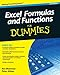Excel Formulas and Functions For Dummies (For Dummies (Computer/Tech))Put the power of Excel formulas and functions to work for you!Excel is a complex program. Mastering the use of formulas and functions lets you use Excel to compute useful day ...Only \$5.38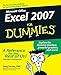Excel 2007 For DummiesOne look at Excel 2007, with its new Office Button, Quick Access toolbar, and Ribbon, and you realize you’re not in Kansas anymore. Well, have no fear— Excel 2007 for Dummies ...Only \$1.16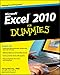Excel 2010 For DummiesThe bestselling Excel book on the market, updated for Excel 2010As the world's leading spreadsheet application, Excel has a huge user base. The release of Office 2010 brings ...\$16.99  \$14.0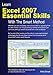Learn Excel 2007 Essential Skills with the Smart Method: Courseware Tutorial for Self ...This book is very, very different to any other computer book you have ever read. The Smart Method provides an entirely new and better way to learn Excel. Here are just five of ...Only \$13.48

### Links to my other pages

##### My other IT-related pages

How to center your webpage horizontally and vertically using CSS

The perfect Christmas gifts for web developers and designers

Updated: 12/05/2012, WebaliciousGuides
3

Only logged-in users are allowed to comment. Logindustytoes on 10/06/2011

Wow, this is WAY over my head, but I am sure it will be a great help to many others who use Excel.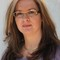nightowl on 10/04/2011

#### You might also like

How To Use Basic Functions In GIMP For Beginners

GIMP is a free image manipulation program which can be intimidating at first,...

How To Cut Out Parts Of A Picture In GIMP For Beginners

Along with making something transparent, cutting an element out of an image a...

Disclosure: This page generates income for authors based on affiliate relationships with our partners, including Amazon, Google and others.